When you type a “=” and a letter inside an Excel cell, all the available formulas come up for you to choose. There are tons of built-in formulas in Excel, many of which you’ll use over and over. These built-in functions have been optimized by Microsoft developers, so their performance is quick and reliable.

However, sometimes there simply aren’t any formulas to do exactly what you need. Other times, there are formulas, but the nested functions are way too complex. Take this Excel formula for instance:

``=IF(AND(SIGN(A1)=1, SIGN(B1) = 1), SUM(A1:B1), IF(A1>0, A1, IF(B1>0, B1)))``

All this lengthy formula does it adds the positive numbers in cells A1 and B1. It’s not flexible at all, so you’re stuck with a two-member row vector unless you substantially modify the formula.

Keep in mind this formula isn’t really even that complicated. It only looks at four cases (A&B>0, A>0, B>0, A&B<0). If you have three rows, you end up with eight cases (23), since each column can be negative or positive and we have three columns. 2x grows very quickly, and you certainly don’t want to write a formula to test all those scenarios.

To solve this problem, and make understanding your calculations much easier, you can write lengthy logic like this into code without needing to nest various built-in Excel functions. When you create your own function in Excel using VBA, it is called a user defined function, or UDF. VBA user defined functions are extremely powerful and they can make your Excel spreadsheets far more userful. I’ve included several of my favorite VBA UDFs in my Excel VBA Examples page.

## Defining the Function

Let’s talk about how to build our VBA user defined functions. The first thing we need to do is define our function by giving it a name, the input parameters, and the intended output. We very briefly talked about the difference between statements and functions before, so you may be able to guess how subroutines and functions differ. But let’s make this explicit.

### Subroutines and Functions

The main difference is that subroutines have no return value. This means that when we call a subroutine, nothing is required to pop out at the end. With functions, though, there is some value (even if it is `Null`) that must be output. At the end of your function, the function name is set to some value.

Another important difference is that functions generally take inputs, while subs might not. Due to this input requirement, functions cannot be run independently like subroutines.

We can always open our Macros dialogue box and run a subroutine (assuming it has no input requirements), but functions, including VBA user defined functions, cannot be run like this. Conversely, functions can be placed in cells as formulas, whereas subroutines cannot. Functions basically take inputs, process them, and return some result, while subroutines perform actions and calculations, but they do not return values like functions do.

### The Function Name and its Return Type

The first step to creating your own VBA user defined function is creating a function name. We might want to specify an output type, as well, to ensure data integrity later. We’ll explain what that means momentarily.

For the name, you can use anything you’d like. The only caveat here is to avoid naming two functions the same thing. You can have two functions with the same name by placing them in two different modules, but it can easily be confusing for someone (including yourself) trying to figure out the program later.

For example, you might have my_function in Area_Module and my_function in Perimeter_Module, and you can call them in your spreadsheet like:

``=Area_Module.my_function()``

or

``=Perimeter_Module.my_function()``

Each one will properly reference its respective code, but this is bad form and simply just a bad idea. Play it safe and just don’t name your functions the same.

It is also advisable to avoid using the same name as built-in Excel functions, as Excel will choose its own function over yours.

To declare a function, you simply start your VBA user defined function by opening the Visual Basic editor and typing something like this:

``````Function my_function()
'...
End Function``````

Make powerful macros with our free VBA Developer Kit

Tutorials like this can be complicated. That’s why we created our free VBA Developer Kit and our Big Book of Excel VBA Macros to supplement this tutorial. Grab them below and you’ll be writing powerful macros in no time.

I'll take a free VBA Developer Kit

Notice the format is exactly the same as the subroutine declarations you’re used to, except we use the `Function` keyword to alert VBA that we have a function, not a sub.

#### The Return Type

Now that you have a function name, you can determine its return type in the naming line. The “Return Type” just means how you want the output value to be stored. This is as simple as dimensioning a normal variable using the `As` syntax.

For example, we might have:

``````Function my_first_UDF() As Double
'...
End Function``````

See the similarities between that and using a Dim statement to dimensionalize a variable?

You can change the As Double to any VBA variable type. If you omit it, the default return type will be `Variant`. If you know your output should always be a certain type, it is better to ensure consistency by requiring the output be entered explicitly. That way, you can catch errors earlier. You certainly don’t want a function that calculates area to output a string (even if it is a number typed as `String`), because you might not notice the problem until one of your users sends an email complaining about it. I may or may not be speaking from experience…

#### Return Values

To return the value calculated in the function, you just equate the function with the value:

``````Function my_reformed_UDF_95() As Double
my_reformed_UDF_95 = 15.17
End Function``````

Whenever my_reformed_UDF_95 is called, it will give back the number 15.17. This short VBA user defined function example illustrates how to set the function equal to a value. You simply set your function name equal to a value.

## Input Variables

The input variables, or arguments, for the function are the second step in construction. Once we have a name (and perhaps a variable type), we can look at passing our function some inputs.

Best Practices: It’s a good idea to send data to your function as arguments rather than using hardcoded variables or cell references. It makes modifying everything much easier, and it allows for greater portability.

### Required Arguments

Most functions will contain at least one required argument. Let’s start by building ourselves a geometric Area function using VBA. Calculating an area is simple and illustrates VBA UDF concepts rather well.

We will look at both triangles and rectangles. For both, we have two required inputs, the base and the height.

To force our users to enter arguments, we simply list them between the parentheses in the naming line of the function, like this:

``Function get_area(base As Double, height As Double) As Double``

When we call this function, we should see the required inputs, like this: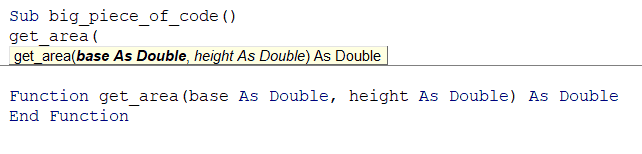Intellisense displays the required inputs for our VBA user defined function

The argument fields won’t appear when you type the formula in Excel, but you can make the names of your arguments show up by pressing Ctrl+Shift+A. This is a neat little trick many people don’t know.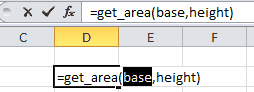List user defined function arguments in Excel with Ctrl+Shift+A

### Optional Arguments

Sometimes it’s beneficial to allow our users to use optional arguments. When writing the function name and inputs, we must list all optional arguments last, but they’re easy to declare. We just use the `Optional` keyword.

An optional argument, such as “type of shape” might look like this:

``Function get_area(base As Double, height As Double, Optional type_of_shape As String) As Double``

When we call it, we even get the added square brackets, symbolizing the argument isn’t required, from Intellisense: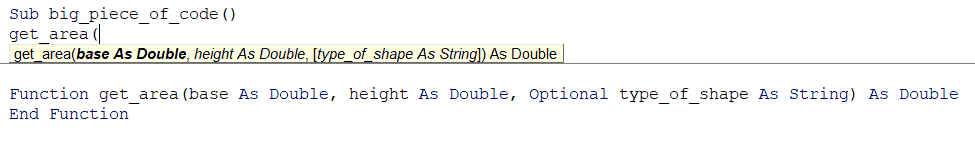Intellisense displays the required and optional inputs for our VBA user defined function

### Default Values

For optional arguments, you might still require some value for code execution. Our area function can be rewritten like this to include a default value for the type_of_shape variable:

``````Function get_area(base As Double, height As Double, Optional type_of_shape As String = "rectangle") As Double

Select Case type_of_shape
Case "rectangle"
get_area = base * height

Case "triangle"
get_area = base * height * 0.5
End Select

End Function``````

We have three possible cases here:

1. The user enters `get_area(10,15)`
2. The user enters `get_area(10,15,"rectangle")`
3. The user enters `get_area(10,15,"triangle")`

The first two will return 150 while the third one will return 75. Because our default value for type_of_shape is “rectangle”, the rectangle case is executed unless the user specifically passes the string “triangle” for the optional argument.

If you’re using a string input in your user defined function, you may want to convert the variable to UCase inside your VBA Select Case structure to make sure the users input is processed properly regardless of how he/she enters it. You can also consider using the StrComp function or specifying a default output when the user enters an unavailable type into type_of_shape (like “circle”).

### Unknown Number of Arguments

The Area function above is great for shapes that have at least three sides, but what about circles? The formula for a circle’s area is pi * r * r, which only has one argument. We could work around this by entering the radius twice (once for “height”, once for “base”) and adding a “circle” case to the list (`get_area = base * height * pi`).

Another approach is to make the second parameter optional, like this:

``Function get_area(base As Double, Optional height As Double, Optional type_of_shape As String = "rectangle") As Double``

but this might be confusing to our users, who will see `get_area(base as Double, [height as Double], [type_of_shape as String])` in their Intelliense. Does this mean the height is optional all the time? We don’t want our users getting confused.

A better way to deal with this is by using `ParamArray` for the function’s arguments.

``````Function get_area2(type_of_shape As String, ParamArray dimensions())

type_of_shape = LCase(type_of_shape)

Select Case type_of_shape
Case "rectangle"
get_area2 = dimensions(0) * dimensions(1)

Case "triangle"
get_area2 = dimensions(0) * dimensions(1) * 0.5

Case "circle"
get_area2 = dimensions(0) * dimensions(0) * 3.14

End Select

End Function``````

Now the user can enter `get_area2("circle", 5)` or `get_area2("triangle", 5, 10)` to calculate the area of a circle with radius 5 or a triangle with height and base of 5 and 10. The user can enter just enough information as needed. There is no need for confusing optional arguments or weird workarounds.

## Working with Ranges

So far, everything we’ve input and returned has been a single variable (doubles, but could be integers, strings, single objects). What if we want to return an array? What if we want to output that array to a range?

The mess of nested functions we had at the beginning could more easily be calculated via a concise user-defined function.

To send an array (in the form of a bunch of cells) to a function, you just need to declare the variable type in the input as `Range`, like this:

``Function return_sum_of_positives(inputs As Range)``

Now, we can enter something like `=return_sum_of_positives(A1:D23)` into a cell and use the entire range of cells for our calculations.

The nested formulas we had at the beginning were meant to sum positive numbers and ignore negative numbers. We can do this by marching down each row and summing the numbers in the columns if and only if they are greater than 0. The whole code block would look like this:

``````Function return_sum_of_positives(inputs As Range) As Variant
'check the number of rows and columns
num_rows = inputs.Rows.Count
num_cols = inputs.Columns.Count

input_arr = inputs  'make the inputs a local variable

ReDim output_arr(1 To num_rows)  'create a temporary array to hold the outputs

For curr_row = 1 To num_rows  'proceed by looking at each row
row_sum = 0
For curr_col = 1 To num_cols  'sum every column in that row
curr_number = input_arr(curr_row, curr_col)
If curr_number > 0 Then
row_sum = row_sum + curr_number
End If
Next curr_col
output_arr(curr_row) = row_sum
Next curr_row

'since VBA stores things in rows, you need to transpose it for a vertical output
return_sum_of_positives = Application.Transpose(output_arr)

End Function``````

One thing to note is the function’s type: `Variant`. If you try to variable-type it as anything else, you will get an error.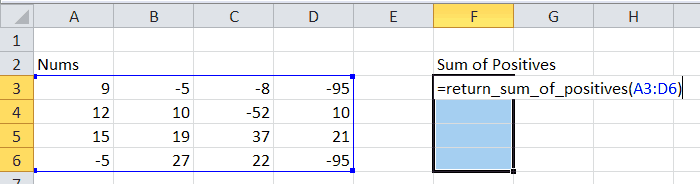The UDF being entered, with the correct number of cells highlighted for the output

If we select F3 through F6 in the picture above and type our formula, we could press `Ctrl+Shift+Enter` to sum up the positive numbers in each row. Without selecting each cell, we would only get a single cell (and only the first number of the array will display). Technically the others are there, but they are hidden behind it - kind of like a 3rd dimension that extends into the screen. We just can’t see its values behind the top one. However you want to picture it, that’s not how we want our function to behave.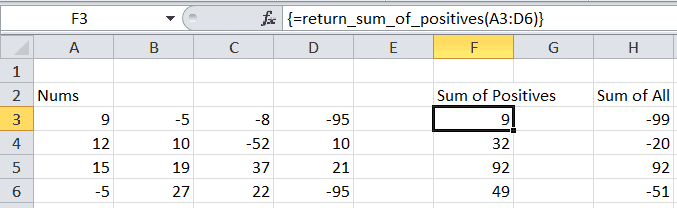We can compare the sums of the rows and our output

The curly braces `{}` appear once you press Ctrl+Shift+Enter. These braces prove that the formula in these cells (F3:F6) are actually part of an array function and they cannot be modified individually.

The VBA user defined function we just created is completely dynamic, unlike the nested formula at the top of this page. We can test A1:B1 or we can test C3:J99 or anything we want. That’s the power of UDFs.

## Conclusion

Writing your own Excel user defined functions with VBA has a lot of advantages. For starters, you can avoid writing complex formulas directly into cells. You can also make sure formulas for basic calculations you find yourself doing over and over. For example, I find myself comparing values in cells all the time, so I write a function to compare cells in Excel.

Once you’ve created your own UDF masterpiece, you can add a description your user defined function by using the VBA MacroOptions feature. These descriptions will appear with your UDF in the Excel Function Wizard dialog box. Once you set it up, all you have to do is click the little fx button beside the formula bar to get a description of what your custom function does and what each variable is.

After this tutorial, you should be able to write your own VBA UDFs to return a single number and functions that return arrays (using the `Ctrl+Shift+Enter` method). You can also take single numbers as arguments, create optional arguments, accept arrays as arguments, and accept a variable number of arguments. We covered a lot in this tutorial, didn’t we?

As stated earlier, it’s still better to use built-in functions if they’re available for your purposes, since they’re already highly optimized to work with Office and Windows. But whenever you need to do something that is not included in the standard set of functions, you can now blaze your own path.

Well, I hope you enjoyed this one. When you’re ready to take your VBA to the next level, subscribe using the form below.